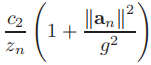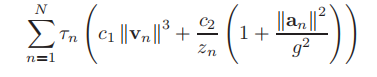# Cannot perform the operation: {convex} .* {convex}.What should I do?Here,z and a are variables,so how to express it?

quad_over_lin Sum of squares over linear.
Z=quad_over_lin(X,Y), where X is a vector and Y is a scalar, is equal to
SUM(ABS(X).^2)./Y if Y is positive, and +Inf otherwise. Y must be real.

``````If X is a matrix, quad_over_lin(X,Y) is a row vector containing the values
of quad_over_lin applied to each column. If X is an N-D array, the operation
is applied to the first non-singleton dimension of X.

quad_over_lin(X,Y,DIM) takes the sum along the dimension DIM of X.
A special value of DIM == 0 is accepted here, which is automatically
replaced with DIM == NDIMS(X) + 1. This has the effect of eliminating
the sum; thus quad_over_lin( X, Y, NDIMS(X) + 1 ) = ABS( X ).^2 ./ Y.

In all cases, Y must be compatible in the same sense as ./ with the squared
sum; that is, Y must be a scalar or the same size as SUM(ABS(X).^2,DIM).

Disciplined convex programming information:
quad_over_lin is convex, nonmontonic in X, and nonincreasing in Y.
Thus when used with CVX expressions, X must be convex (or affine)
and Y must be concave (or affine).``````

I have tried,but it shows “The first argument must be affine."

Formulate the numerator as a sum of squares of an affine argument. I.e., `norm(x)^2 = x'*x`.

a is a matrix with size(2,N),and z is (1,N),and the aim is summation.

What is a_n? is that an individual row or column of a?

The first step for you is to write out what you want in “regular” MATLAB, i.e., if all the inputs are numerically populated double precision variables. Once you have that correct, then you need to formulate it in accordance with CVX’s rules.

It is an individual column of a.I see it on a paper,which says it is convex.

Did you read the help for `quad_over_lin` in my first post?

Sorry,I made a mistake.I see.Thank you very much!

Hello, I think you and I are writing the same thing. I want to ask you what the first half of the formula you posted, the V (n) norm to the third power, represents? Thank youThis?

Maybe you can use pow_pos()

yes，that is the formula.I’m using the term pow_pos,but v is a variable of (2,N),norm to the third power and pow_pos will give you an error to say that you can’t use internally convex.

Which are variables in this formula?

three v(2,N) a(2,N) and z(1,N)
But I dont know the variable of a and v are (2,N).

use norms,quad_over_lin and pow_pos,maybe you can solve.

Ok.but I’m not sure the variable of a and v are (2,N) or (1,N)
How do you think it should be expressed

If it is 2 dimentions,it should be (2,N)